# Section 21.2. Producing Image Data

### 21.2. Producing Image Data

There are two approaches to generating image data. The high-level method is to treat the image as a drawing surface and use the methods of Graphics2D to render things into the image. The second way is to twiddle the bits that represent the pixels of the image data yourself. This is harder, but gives you arbitrary control for handling specific formats or mathematically analyzing or creating image data.

#### 21.2.1. Drawing Animations

Let's begin with the simpler approach, rendering on an image through drawing. We'll throw in a twist to make things interesting: we'll build an animation. Each frame will be rendered as we go along. This is very similar to the double buffering we examined in the last chapter, but this time we'll use a timer, instead of mouse events, as the signal to generate new frames.

Swing performs double buffering automatically, so we don't even have to worry about the animation flickering. Although it looks like we're drawing directly to the screen, we're really drawing into an image that Swing uses for double buffering. All we need to do is draw the right thing at the right time.

Let's look at an example, Hypnosis, that illustrates the technique. This example shows a constantly shifting shape that bounces around the inside of a component. When screen savers first came of age, this kind of thing was pretty hot stuff. Hypnosis is shown in Figure 21-2.

##### Figure 21-2. A simple animation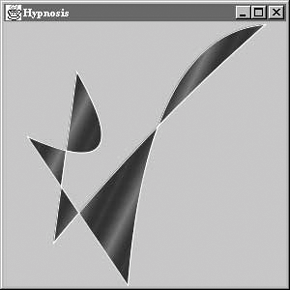Here is its source code:

`     //file: Hypnosis.java     import java.awt.*;     import java.awt.event.*;     import java.awt.geom.GeneralPath;     import javax.swing.*;     public class Hypnosis extends JComponent implements Runnable {       private int[] coordinates;       private int[] deltas;       private Paint paint;       public Hypnosis(int numberOfSegments) {         int numberOfCoordinates = numberOfSegments * 4 + 2;         coordinates = new int[numberOfCoordinates];         deltas = new int[numberOfCoordinates];         for (int i = 0 ; i < numberOfCoordinates; i++) {           coordinates[i] = (int)(Math.random(  ) * 300);           deltas[i] = (int)(Math.random(  ) * 4 + 3);           if (deltas[i] > 4) deltas[i] = -(deltas[i] - 3);         }         paint = new GradientPaint(0, 0, Color.blue,             20, 10, Color.red, true);         Thread t = new Thread(this);         t.start(  );       }       public void run(  ) {         try {           while (true) {             timeStep(  );             repaint(  );             Thread.sleep(1000 / 24);           }         }         catch (InterruptedException ie) {}       }       public void paint(Graphics g) {         Graphics2D g2 = (Graphics2D)g;         g2.setRenderingHint(RenderingHints.KEY_ANTIALIASING,             RenderingHints.VALUE_ANTIALIAS_ON);         Shape s = createShape(  );         g2.setPaint(paint);         g2.fill(s);         g2.setPaint(Color.white);         g2.draw(s);       }       private void timeStep(  ) {         Dimension d = getSize(  );         if (d.width == 0 || d.height == 0) return;         for (int i = 0; i < coordinates.length; i++) {           coordinates[i] += deltas[i];           int limit = (i % 2 == 0) ? d.width : d.height;           if (coordinates[i] < 0) {             coordinates[i] = 0;             deltas[i] = -deltas[i];           }           else if (coordinates[i] > limit) {             coordinates[i] = limit - 1;             deltas[i] = -deltas[i];           }         }       }       private Shape createShape(  ) {         GeneralPath path = new GeneralPath(  );         path.moveTo(coordinates, coordinates);         for (int i = 2; i < coordinates.length; i += 4)           path.quadTo(coordinates[i], coordinates[i + 1],               coordinates[i + 2], coordinates[i + 3]);         path.closePath(  );         return path;       }       public static void main(String[] args) {         JFrame frame = new JFrame("Hypnosis");         frame.getContentPane(  ).add( new Hypnosis(4) );         frame.setSize(300, 300);         frame.setDefaultCloseOperation( JFrame.EXIT_ON_CLOSE );         frame.setVisible(true);       }     } `

The main( ) method does the usual grunt work of setting up the JFrame that holds our animation component.

The Hypnosis component has a very basic strategy for animation. It holds some number of coordinate pairs in its coordinates member variable. A corresponding array, deltas, holds "delta" amounts that are added to the coordinates each time the figure is supposed to change. To render the complex shape you see in Figure 21-2, Hypnosis creates a special Shape object from the coordinate array each time the component is drawn.

Hypnosis's constructor has two important tasks. First, it fills up the coordinates and deltas arrays with random values. The number of array elements is determined by an argument to the constructor. The constructor's second task is to start up a new thread that drives the animation.

The animation is done in the run( ) method. This method calls timeStep( ), which repaints the component and waits for a short time (details to follow). Each time timeStep( ) is called, the coordinates array is updated and repaint( ) is called. This results in a call to paint( ), which creates a shape from the coordinate array and draws it.

The paint( ) method is relatively simple. It uses a helper method, called createShape( ), to create a shape from the coordinate array. The shape is then filled, using a Paint stored as a member variable. The shape's outline is also drawn in white.

The timeStep( ) method updates all the elements of the coordinate array by adding the corresponding element of deltas. If any coordinates are now out of the component's bounds, they are adjusted, and the corresponding delta is negated. This produces the effect of bouncing off the sides of the component.

createShape( ) creates a shape from the coordinate array. It uses the GeneralPath class, a useful Shape implementation that allows you to build shapes using straight and curved line segments. In this case, we create a shape from a series of quadratic curves, close it to create an area, and fill it.

#### 21.2.2. BufferedImage Anatomy

So far, we've talked about java.awt.Images and how they can be loaded and drawn. What if you really want to get inside the image to examine and update its data? Image doesn't give you access to its data. You'll need to use a more sophisticated kind of image: java.awt.image.BufferedImage. The classes are closely relatedBufferedImage, in fact, is a subclass of Image. BufferedImage gives you all sorts of control over the actual data that makes up the image and provides many capabilities beyond the basic Image class. Because it's a subclass of Image, you can still pass a BufferedImage to any of Graphics2D's methods that accept an Image.

To create an image from raw data, you need to understand exactly how a BufferedImage is put together. The full details can get quite complexthe BufferedImage class was designed to support images in nearly any storage format you can imagine. But, for common operations, it's not that difficult to use. Figure 21-3 shows the elements of a BufferedImage.

##### Figure 21-3. Inside a BufferedImage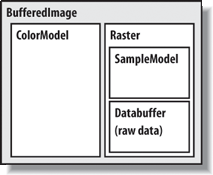An image is simply a rectangle of colored pixels, which is a simple enough concept. There's a lot of complexity underneath the BufferedImage class, because there are a lot of different ways to represent the colors of pixels. You might have, for instance, an image with RGB data in which each pixel's red, green, and blue values were stored as the elements of byte arrays. Or you might have an RGB image where each pixel was represented by an integer that contained red, green, and blue component values. Or you could have a 16-level grayscale image with eight pixels stored in each element of an integer array. You get the idea; there are many different ways to store image data, and BufferedImage is designed to support all of them.

A BufferedImage consists of two pieces, a Raster and a ColorModel. The Raster contains the actual image data. You can think of it as an array of pixel values. It can answer the question, "What are the color data values for the pixel at 51, 17?" The Raster for an RGB image would return three values, while a Raster for a grayscale image would return a single value. WritableRaster, a subclass of Raster, also supports modifying pixel data values.

The ColorModel's job is to interpret the image data as colors. The ColorModel can translate the data values that come from the Raster into Color objects. An RGB color model, for example, would know how to interpret three data values as red, green, and blue. A grayscale color model could interpret a single data value as a gray level. Conceptually, at least, this is how an image is displayed on the screen. The graphics system retrieves the data for each pixel of the image from the Raster. Then the ColorModel tells what color each pixel should be, and the graphics system is able to set the color of each pixel.

The Raster itself is made up of two pieces: a DataBuffer and a SampleModel. A DataBuffer is a wrapper for the raw data arrays, which are byte, short, or int arrays. DataBuffer has handy subclasses, DataBufferByte, DataBufferShort, and DataBufferInt, that allow you to create a DataBuffer from raw data arrays. You'll see an example of this technique later in the StaticGenerator example.

The SampleModel knows how to extract the data values for a particular pixel from the DataBuffer. It knows the layout of the arrays in the DataBuffer and is ultimately responsible for answering the question, "What are the data values for pixel x, y?" SampleModels are a little tricky to work with, but, fortunately, you'll probably never need to create or use one directly. As we'll see, the Raster class has many static ("factory") methods that create preconfigured Rasters for you, including their component DataBuffers and SampleModels.

As Figure 21-1 shows, the 2D API comes with various flavors of ColorModels, SampleModels, and DataBuffers. These serve as handy building blocks that cover most common image storage formats. You'll rarely need to subclass any of these classes to create a BufferedImage.

#### 21.2.3. Color Models

As we've said, there are many different ways to encode color information: red, green, blue (RGB) values; hue, saturation, value (HSV); hue, lightness, saturation (HLS); and more. In addition, you can provide full-color information for each pixel, or you can just specify an index into a color table (palette) for each pixel. The way you represent a color is called a color model. The 2D API provides tools to support any color model you could imagine. Here, we'll just cover two broad groups of color models: direct and indexed.

As you might expect, you must specify a color model in order to generate pixel data; the abstract class java.awt.image.ColorModel represents a color model. By default, Java 2D uses a direct color model called ARGB. The A stands for "alpha," which is the historical name for transparency. RGB refers to the red, green, and blue color components that are combined to produce a single, composite color. In the default ARGB model, each pixel is represented by a 32-bit integer that is interpreted as four 8-bit fields; in order, the fields represent the alpha (transparency), red, green, and blue components of the color, as shown in Figure 21-4.

##### Figure 21-4. ARGB color encoding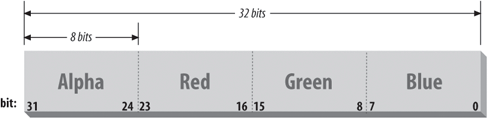To create an instance of the default ARGB model, call the static getRGBdefault( ) method in ColorModel. This method returns a DirectColorModel object; DirectColorModel is a subclass of ColorModel. You can also create other direct color models by calling a DirectColorModel constructor, but you shouldn't need to unless you have a fairly exotic application.

In an indexed color model, each pixel is represented by a smaller piece of information: an index into a table of real color values. For some applications, generating data with an indexed model may be more convenient. If you are writing an application for an 8-bit display or smaller, using an indexed model may be more efficient, because your hardware is internally using an indexed color model of some form.

#### 21.2.4. Creating an Image

Let's take a look at producing some image data. A picture is worth a thousand words, and, fortunately, we can generate a pretty picture in significantly fewer than a thousand words of Java. If we just want to render image frames byte by byte, you can put together a BufferedImage pretty easily.

The following application, ColorPan, creates an image from an array of integers holding RGB pixel values:

`     //file: ColorPan.java     import java.awt.*;     import java.awt.image.*;     import javax.swing.*;     public class ColorPan extends JComponent {       BufferedImage image;       public void initialize(  ) {         int width = getSize(  ).width;         int height = getSize(  ).height;         int[] data = new int [width * height];         int i = 0;         for (int y = 0; y < height; y++) {           int red = (y * 255) / (height - 1);           for (int x = 0; x < width; x++) {             int green = (x * 255) / (width - 1);             int blue = 128;             data[i++] = (red << 16) | (green << 8 ) | blue;           }         }         image = new BufferedImage(width, height,             BufferedImage.TYPE_INT_RGB);         image.setRGB(0, 0, width, height, data, 0, width);       }       public void paint(Graphics g) {         if (image == null)             initialize(  );         g.drawImage(image, 0, 0, this);       }       public void setBounds(int x, int y, int width, int height) {         super.setBounds(x,y,width,height);         initialize(  );       }       public static void main(String[] args) {         JFrame frame = new JFrame("ColorPan");         frame.getContentPane(  ).add(new ColorPan(  ));         frame.setSize(300, 300);         frame.setDefaultCloseOperation( JFrame.EXIT_ON_CLOSE );         frame.setVisible(true);       }     } `

Give it a try. The size of the image is determined by the size of the application window. You should get a very colorful box that pans from deep blue at the upper-left corner to bright yellow at the bottom right, with green and red at the other extremes.

We create a BufferedImage in the initialize( ) method and then display the image in paint( ). The variable data is a 1D array of integers that holds 32-bit RGB pixel values. In initialize( ), we loop over every pixel in the image and assign it an RGB value. The blue component is always 128, half its maximum intensity. The red component varies from 0 to 255 along the y-axis; likewise, the green component varies from 0 to 255 along the x-axis. This statement combines these components into an RGB value:

`     data[i++] = (red << 16) | (green << 8 ) | blue; `

The bitwise left-shift operator (<<) should be familiar to anyone who has programmed in C. It simply shoves the bits over by the specified number of positions in our 32-bit value.

When we create the BufferedImage, all its data is zeroed out. All we specify in the constructor is the width and height of the image and its type. BufferedImage includes quite a few constants representing image storage types. We've chosen TYPE_INT_RGB here, which indicates we want to store the image as RGB data packed into integers. The constructor takes care of creating an appropriate ColorModel, Raster, SampleModel, and DataBuffer for us. Then, we simply use the setRGB( )method to assign our data to the image. In this way, we've side-stepped the messy innards of BufferedImage. In the next example, we'll take a closer look at the details.

Once we have the image, we can draw it on the display with the standard drawImage( ) method. We also override the Component setBounds( ) method in order to determine when the frame is resized and reinitialize the drawing image to the new size.

#### 21.2.5. Updating a BufferedImage

BufferedImage can also be used to update an image dynamically. Because the image's data arrays are directly accessible, you can simply change the data and redraw the picture whenever you want. This is probably the easiest way to build your own low-level animation software. The following example simulates the static on an old black-and-white television screen. It generates successive frames of random black and white pixels and displays each frame when it is complete. Figure 21-5 shows one frame of random static.

##### Figure 21-5. A frame of random static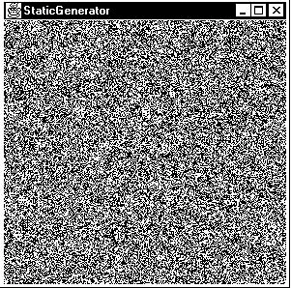Here's the code:

`     //file: StaticGenerator.java     import java.awt.*;     import java.awt.event.*;     import java.awt.image.*;     import java.util.Random;     import javax.swing.*;     public class StaticGenerator extends JComponent implements Runnable {       byte[] data;       BufferedImage image;       Random random;       public void initialize(  ) {         int w = getSize(  ).width, h = getSize(  ).height;         int length = ((w + 7) * h) / 8;         data = new byte[length];         DataBuffer db = new DataBufferByte(data, length);         WritableRaster wr = Raster.createPackedRaster(db, w, h, 1, null);         ColorModel cm = new IndexColorModel(1, 2,             new byte[] { (byte)0, (byte)255 },             new byte[] { (byte)0, (byte)255 },             new byte[] { (byte)0, (byte)255 });         image = new BufferedImage(cm, wr, false, null);         random = new Random(  );       }       public void run(  ) {         if ( random == null )             initialize(  );         while (true) {           random.nextBytes(data);           repaint(  );           try { Thread.sleep(1000 / 24); }           catch( InterruptedException e ) { /* die */ }         }       }       public void paint(Graphics g) {         if (image == null) initialize(  );         g.drawImage(image, 0, 0, this);       }       public void setBounds(int x, int y, int width, int height) {         super.setBounds(x,y,width,height);         initialize(  );       }       public static void main(String[] args) {         //RepaintManager.currentManager(null).setDoubleBufferingEnabled(false);         JFrame frame = new JFrame("StaticGenerator");         StaticGenerator staticGen = new StaticGenerator(  );         frame.getContentPane(  ).add( staticGen );         frame.setSize(300, 300);         frame.setDefaultCloseOperation( JFrame.EXIT_ON_CLOSE );         frame.setVisible(true);         new Thread( staticGen ).start(  );       }     } `

The initialize( ) method sets up the BufferedImage that produces the sequence of images. We build this image from the bottom up, starting with the raw data array. Since we're only displaying two colors here, black and white, we need only one bit per pixel. We want a 0 bit to represent black and a 1 bit to represent white. This calls for an indexed color model, which we'll create a little later.

We'll store our image data as a byte array, where each array element holds eight pixels from our black-and-white image. The array length, then, is calculated by multiplying the width and height of the image and dividing by eight. To keep things simple, we'll arrange for each image row to start on a byte boundary. For example, an image 13 pixels wide actually uses 2 bytes (16 bits) for each row:

`     int length = (w + 7)/8 * h; `

This calculation rounds upward the number of bytes required to fill a row and then multiplies by the number of rows. Next, the actual byte array is created. The member variable data holds a reference to this array. Later, we'll use data to change the image data dynamically. Once we have the image data array, it's easy to create a DataBuffer from it:

`     data = new byte[length];     DataBuffer db = new DataBufferByte(data, length); `

DataBuffer has several subclasses, such as DataBufferByte, that make it easy to create a data buffer from raw arrays.

The next step, logically, is to create a SampleModel. We could then create a Raster from the SampleModel and the DataBuffer. Lucky for us, though, the Raster class contains a bevy of useful static methods that create common types of Rasters. One of these methods creates a Raster from data that contains multiple pixels packed into array elements. We simply use this method, supplying the data buffer, the width and height, and indicating that each pixel uses one bit:

`     WritableRaster wr = Raster.createPackedRaster(db, w, h, 1, null/*ul corner*/); `

The last argument to this method is a java.awt.Point that indicates where the upper-left corner of the Raster should be. By passing null, we use the default of 0, 0.

The last piece of the puzzle is the ColorModel. Each pixel is either 0 or 1, but how should that be interpreted as color? In this case, we use an IndexColorModel with a very small palette. The palette has only two entries, one each for black and white:

`     ColorModel cm = new IndexColorModel(1, 2,             new byte[] { (byte)0, (byte)255 },             new byte[] { (byte)0, (byte)255 },             new byte[] { (byte)0, (byte)255 }); `

The IndexColorModel constructor that we've used here accepts the number of bits per pixel (one), the number of entries in the palette (two), and three byte arrays that are the red, green, and blue components of the palette colors. Our palette consists of two colors: black (0, 0, 0) and white (255, 255, 255).

Now that we've got all the pieces, we just need to create a BufferedImage. This image is also stored in a member variable so we can draw it later. To create the BufferedImage, we pass the color model and writable raster we just created:

`     image = new BufferedImage(cm, wr, false, null); `

All the hard work is done now. Our paint( ) method just draws the image, using drawImage( ).

The init( ) method starts a thread that generates the pixel data. The run( ) method takes care of generating the pixel data. It uses a java.util.Random object to fill the data image byte array with random values. Since the data array is the actual image data for our image, changing the data values changes the appearance of the image. Once we fill the array with random data, a call to repaint( ) shows the new image on the screen.

You can also try turning off double buffering by uncommenting the line involving the RepaintManager. Now it will look even more like an old TV screen flickering and all!

That's about all there is. It's worth noting how simple it is to create this animation. Once we have the BufferedImage, we treat it like any other image. The code that generates the image sequence can be arbitrarily complex. But that complexity never infects the simple task of getting the image on the screen and updating it.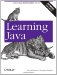Learning Java
ISBN: 0596008732
EAN: 2147483647
Year: 2005
Pages: 262

Similar book on Amazon

flylib.com © 2008-2017.
If you may any questions please contact us: flylib@qtcs.net